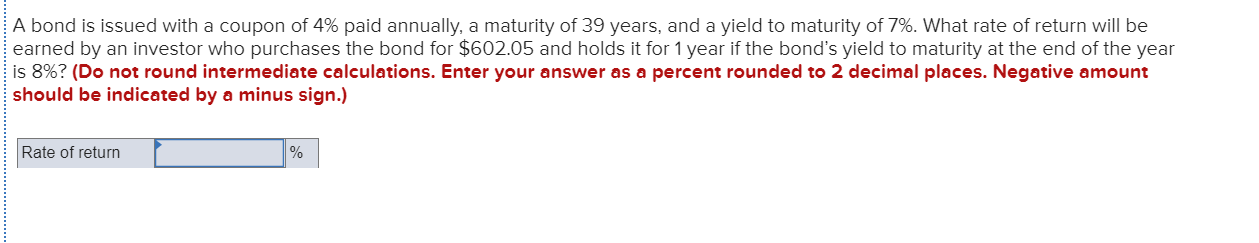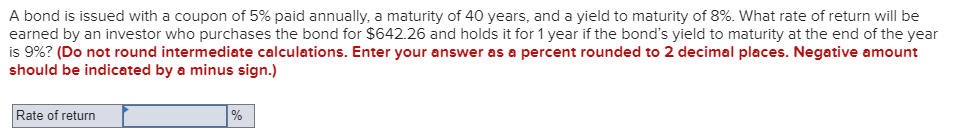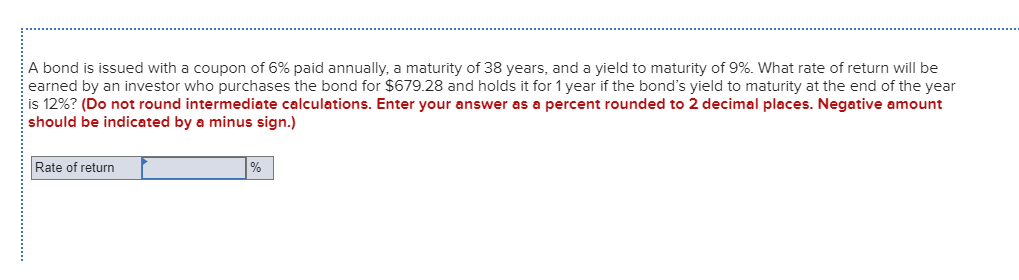Question

# Suppose that a company issues a bond with a coupon of 4% paid annually. The bond has a maturity of 30 years and a yield to maturity of 7%. An investor purchased this bond at a fair price and holds the bond for 1 year. If the yield to maturity at the end

Suppose that a company issues a bond with a coupon of 4% paid annually. The bond has a maturity of 30 years and a yield to maturity of 7%. An investor purchased this bond at a fair price and holds the bond for 1 year.

If the yield to maturity at the end of bond’s life changes to 8%, what will be the rate of return that this investor is going to earn at the end of year 1?

The fair price of the bond is simply the present value today of all future cash flows from an investment in a bond

We need at least 10 more requests to produce the answer.

0 / 10 have requested this problem solution

The more requests, the faster the answer.

All students who have requested the answer will be notified once they are available.

#### Earn Coins

Coins can be redeemed for fabulous gifts.

Similar Homework Help Questions
• ### A bond is issued with a coupon of 6% paid annually, a maturity of 34 years,...

A bond is issued with a coupon of 6% paid annually, a maturity of 34 years, and a yield to maturity of 8%. What rate of return will be earned by an investor who purchases the bond for \$768.26 and holds it for 1 year if the bond’s yield to maturity at the end of the year is 9%?

• ### A bond is issued with a coupon of 6% paid annually, a maturity of 35 years,...

A bond is issued with a coupon of 6% paid annually, a maturity of 35 years, and a yield to maturity of 9%. What rate of return will be earned by an investor who purchases the bond for \$683.00 and holds it for 1 year if the bond’s yield to maturity at the end of the year is 10%? (Do not round intermediate calculations. Enter your answer as a percent rounded to 2 decimal places. Negative amount should be indicated...

• ### A bond has a face value of \$1,000, a coupon of 5% paid annually, a maturity...

A bond has a face value of \$1,000, a coupon of 5% paid annually, a maturity of 34 years, and a yield to maturity of 8%. What rate of return will be earned by an investor who purchases the bond for \$652.39 and holds it for 1 year if the bond’s yield to maturity at the end of the year is 9%? (Do not round intermediate calculations. Enter your answer as a percent rounded to 2 decimal places. Negative amount...

• ### A bond is issued with a coupon of 4% paid annually, a maturity of 39 years,...A bond is issued with a coupon of 4% paid annually, a maturity of 39 years, and a yield to maturity of 7%. What rate of return will be earned by an investor who purchases the bond for \$602.05 and holds it for 1 year if the bond's yield to maturity at the end of the year is 8%? (Do not round intermediate calculations. Enter your answer as a percent rounded to 2 decimal places. Negative amount should be indicated...

• ### a.        Suppose you purchase a 20-year,8% coupon bond with a yield to maturity of 10%. For...

a.        Suppose you purchase a 20-year,8% coupon bond with a yield to maturity of 10%. For a face value of \$1000, what should be the initial price of the bond assuming that the bond is paying interest semi-annually? b.       If the bond’s yield to maturity changes to be 12%, what will its price be five years later? c. If you purchased the bond at THE PRICE YOU COMPUTED AT (a) and sold it 5 years later, what would the rate...

• ### A bond is issued with a coupon of 5% paid annually, a maturity of 40 years,...A bond is issued with a coupon of 5% paid annually, a maturity of 40 years, and a yield to maturity of 8%. What rate of return will be earned by an investor who purchases the bond for \$642.26 and holds it for 1 year if the bond's yield to maturity at the end of the year is 9%? (Do not round intermediate calculations. Enter your answer as a percent rounded to 2 decimal places. Negative amount should be indicated...

• ### Today (T=0), an investor purchased a five year bond with an 8.0% coupon at par. Assume...

Today (T=0), an investor purchased a five year bond with an 8.0% coupon at par. Assume interest rates do not change from now until the bond’s maturity. If the investor holds the bond from now until maturity, the investor’s rate of return will be closest to

• ### Today (T=0), an investor purchased a five year bond with an 8.0% coupon at par. Assume...

Today (T=0), an investor purchased a five year bond with an 8.0% coupon at par. Assume interest rates do not change from now until the bond’s maturity. If the investor holds the bond from now until maturity, the investor’s rate of return will be closest to: A. 4.0% B. 6.7% C. 7.0% D. 8.0% E. 9.7%

• ### URGENT!! A bond is issued with a coupon of 6% paid annually, a maturity of 38...URGENT!! A bond is issued with a coupon of 6% paid annually, a maturity of 38 years, and a yield to maturity of 9%. What rate of return will be earned by an investor who purchases the bond for \$679.28 and holds it for 1 year if the bond's yield to maturity at the end of the year is 12%? (Do not round intermediate calculations. Enter your answer as a percent rounded to 2 decimal places. Negative amount should be...

• ### 1a. Calculate the price of a bond where the coupon rate is 5% (pays annually), the...

1a. Calculate the price of a bond where the coupon rate is 5% (pays annually), the market interest rate is 4%, and the life of the bond is 10 years. 1b. Suppose that you have an annual pay 7-year bond with a price of \$1,100, paying a 4.5% coupon, with a face value of \$1,000. What is the bond’s yield to maturity (YTM)? 1c. A bond sells for \$900 today. Its coupon rate is 3%. The expected price in one...